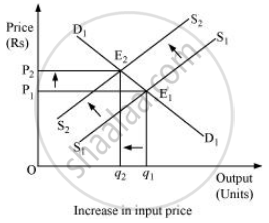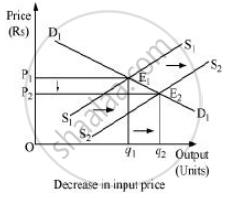Advertisement Remove all ads

# How Do the Equilibrium Price and the Quantity of a Commodity Change When Price of Input Used in Its Production Changes? - Economics

Answer in Brief

How do the equilibrium price and the quantity of a commodity change when price of input used in its production changes?

Advertisement Remove all ads

#### Solution

The change in the price of input alters the cost of production of a commodity.

Let us analyze the two different cases.

1. Increase in input price

If the input price of a firm increases, the cost of production will also increase, which will discourage the firm’s incentive to produce and supply the commodity. This will lead to a left upward shift of the marginal cost curve, which further will lead to a leftward parallel shift of an individual firm’s supply curve and finally a leftward shift of the market supply curve. The demand curve remaining the same, the new equilibrium will occur at E2 with higher equilibrium price (P2) and lower quantity of output (q2).1. Decrease in input price

If an input price of a firm decreases, then the cost of production will also decrease. This will shift the marginal cost curve rightward, which implies that the firm’s supply curve will also shift rightward. Consequently, the market supply curve will shift rightward parallelly from S1S1 to S2S2. Demand curve remaining the same, the new equilibrium will occur at E2 with lower equilibrium price (P2) and higher quantity level of output (q2).Is there an error in this question or solution?
Advertisement Remove all ads

#### APPEARS IN

NCERT Class 12 Economics Introductory Microeconomics
Chapter 5 Market Equilibrium
Exercise | Q 12 | Page 87
Advertisement Remove all ads
Advertisement Remove all ads
Share
Notifications

View all notifications

Forgot password?